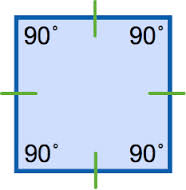# Square

In Geometry, a square is a two-dimensional plane figure with four equal sides and equal angles. In this article, we are going to discuss the definition of the square, properties of square and the terms related to a square and the relationship between them.

## What is a Square?

Square is a regular quadrilateral where all the four sides and angles are equal. All the four angles are right angles. It can also be defined as a rectangle where two adjacent sides have equal length.## Properties of a Square

The most important properties of a square are listed below:

• All four interior angles are equal to 90°
• All four sides of the square are congruent or equal to each other
• The opposite sides of the square are parallel to each other
• The diagonals of the square bisect each other at 90°
• The two diagonals of the square are equal to each other
• The square has 4 vertices and 4 sides
• The diagonal of the square divide it into two similar isosceles triangles.
• Area of the square is equal to the side squared

Area = side2 per square unit

• The perimeter of the square is equal to the sum of all its four sides.

Perimeter = 4 × side of the square

• The length of the diagonals of the square is equal to s√2, where s is the side of the square. As we know, the length of the diagonals is equal to each other. Therefore, by Pythagoras theorem, we can say, diagonal is the hypotenuse and the two sides of the triangle formed by diagonal of the square, are perpendicular and base.

Since, Hypotenuse2 = Base2 + Perpendicular2

Hence, Diagonal2 = Side2 + Side2

Diagonal = $\sqrt{2side^2}$

d = s√2

Where d is the length of the diagonal of a square and s is the side of the square.

## Diagonal of square

It is a segment that connects two opposite vertices of the square. As we have four vertices of a square, thus we can have two diagonals within a square. Diagonals of the square are always greater than its sides.

Below given are some important relation of diagonal of a square and other terms related to the square.

 Relation between Diagonal ‘d’ and side ‘a’ of a square $d = a \sqrt{2}$ Relation between Diagonal ‘d’ and Area ‘A’ of a Square- $d = \sqrt{2A}$ Relation between Diagonal ‘d’ and Perimeter ‘P’ of a Square- $d = \frac{P}{2 \sqrt {2}}$ Relation between Diagonal ‘d’ and Circumradius ‘R’ of a square: d = 2R Relation between Diagonal ‘d’ and diameter of the Circumcircle $d = D_{c}$ Relation between Diagonal ‘d’ and In-radius (r) of a circle- $d = 2\sqrt {2}r$ Relation between Diagonal ‘d’ and diameter of the In-circle $d = \sqrt {2}D_{i}$ Relation between diagonal and length of the segment l- $d = l \frac{2\sqrt {10}}{5}$

### Square Example

Example: Let a square have side equal to 6 cm. Find out its area, perimeter and length of diagonal.

Solution: Given, side of the square, s = 6 cm

Area of the square = s2 = 62 = 36 cm2

Perimeter of the square = 4 ×  s = 4 × 6 cm = 24cm

Length of the diagonal of square = s√2 = 6 × 1.414 = 8.484

Learn more about different geometrical figures here at BYJU’S. Also, download its app to get a visual of such figures and understand the concepts in a more better and creative way.